# Copy cells from the ActiveCell Row to a Database sheet using VBA in Microsoft Excel

In Microsoft Excel, we can copy cells from the activecell row to a specific sheet. In this article we will use VBA code to transfer data from every sheet & merge the data into one worksheet. We will add a Master sheet in the workbook & save the data from the specified range into one sheet.

The example codes will copy to a database sheet with the name Sheet2. Every time we run macro the cells will be placed below the last row with data after the last Column in sheet2. This macro will copy the cells from Column A, D from the ActiveCell.

Following is the image of the sample data: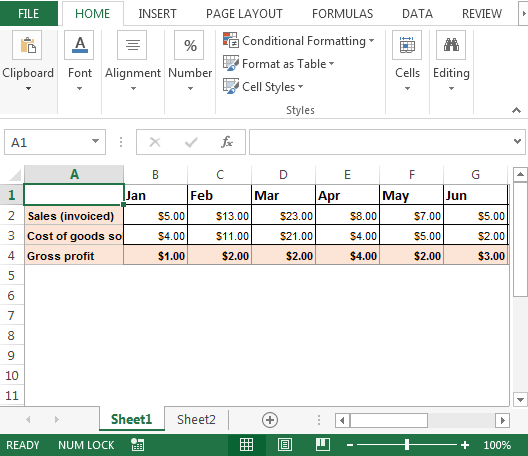To copy cells from activecell row to Sheet2; we need to follow the below steps to launch VB editor:

• Click on Developer tab
• From Code group select Visual Basic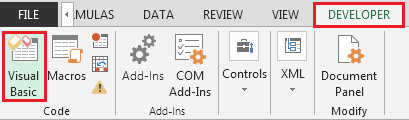• Click on Insert then Module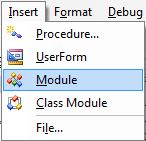• This will create new module
• Enter the following code in the Standard Module

```Sub CopyCells()
Dim sourceRange As Range
Dim destrange As Range
Dim Lr As Long
Lr = LastRow(Sheets("Sheet2")) + 1
Set sourceRange = Sheets("Sheet1").Cells( _
ActiveCell.Row, 1).Range("A1:D1")
Set destrange = Sheets("Sheet2").Range("A" & Lr)
sourceRange.Copy destrange
End Sub
```

```Sub CopyCellsValues()
Dim sourceRange As Range
Dim destrange As Range
Dim Lr As Long
Lr = LastRow(Sheets("Sheet2")) + 1
Set sourceRange = Sheets("Sheet1").Cells( _
ActiveCell.Row, 1).Range("A1:D1")
With sourceRange
Set destrange = Sheets("Sheet2").Range("A" _
& Lr).Resize(.Rows.Count, .Columns.Count)
End With
destrange.Value = sourceRange.Value
End Sub
```

```Function LastRow(sh As Worksheet)
On Error Resume Next
LastRow = sh.Cells.Find(What:="*", _
After:=sh.Range("A1"), _
Lookat:=xlPart, _
LookIn:=xlFormulas, _
SearchOrder:=xlByRows, _
SearchDirection:=xlPrevious, _
MatchCase:=False).Row
On Error GoTo 0
End Function
```

```Function Lastcol(sh As Worksheet)
On Error Resume Next
Lastcol = sh.Cells.Find(What:="*", _
After:=sh.Range("A1"), _
Lookat:=xlPart, _
LookIn:=xlFormulas, _
SearchOrder:=xlByColumns, _
SearchDirection:=xlPrevious, _
MatchCase:=False).Column
On Error GoTo 0
End Function
```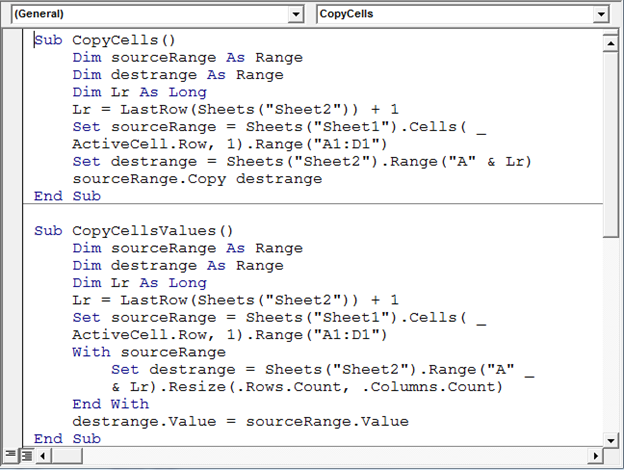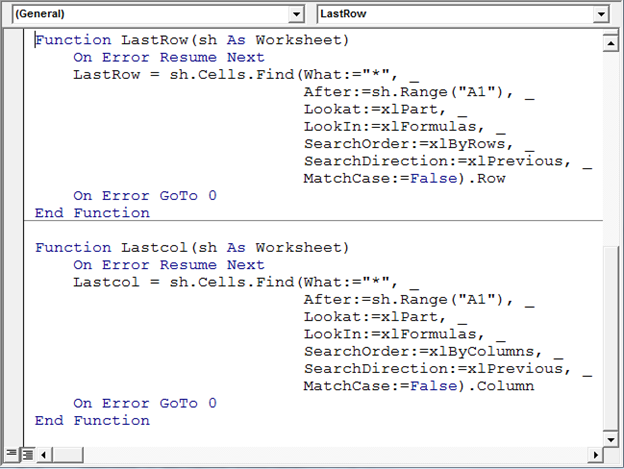• To check the above VBA code; add data in the range “A1:D1” & then run the macro by pressing F5 shortcut key
• The data stored in the specified range will get copied to “Sheet2” starting from A1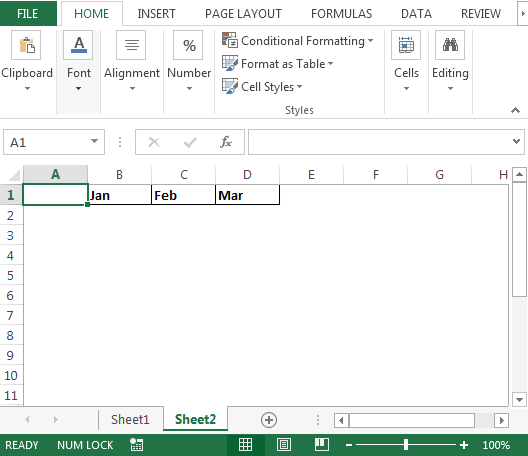• If we run this macro again; the data will saved in the next row; refer below image:• We can change the range in the above macro that suits our requirement

Conclusion: With above macro we can copy cells from the activecell row to a new sheet using VBA code.